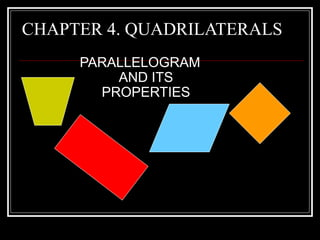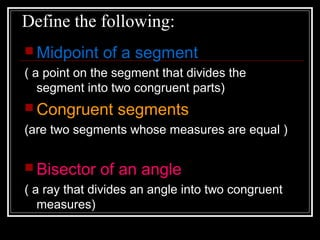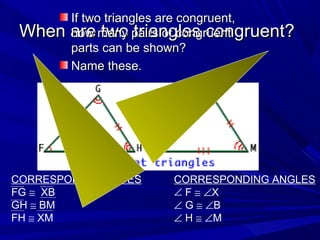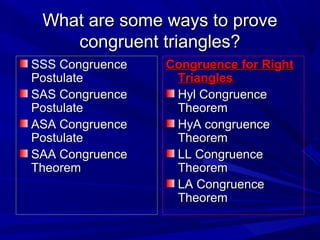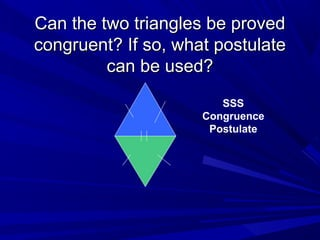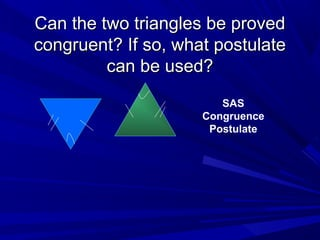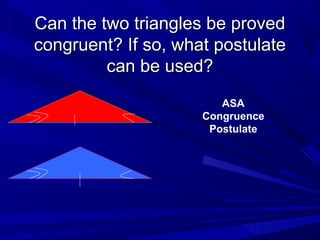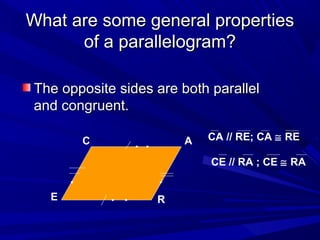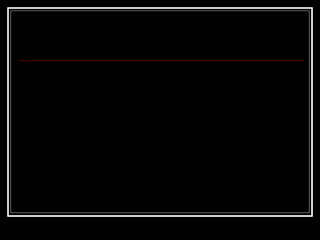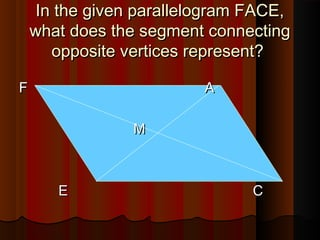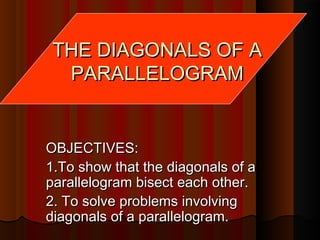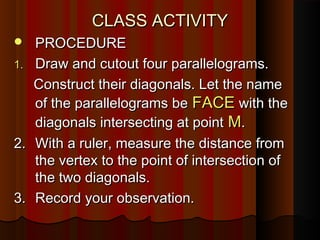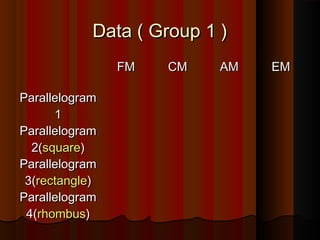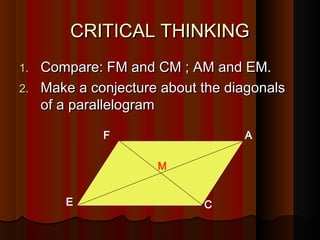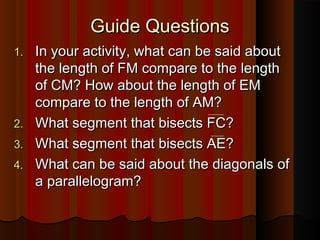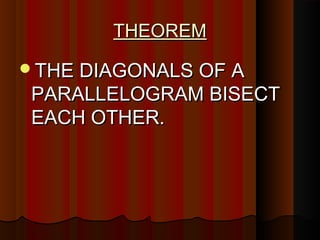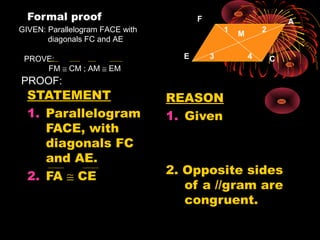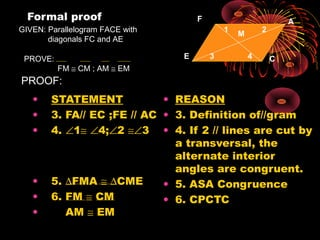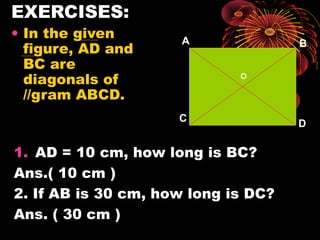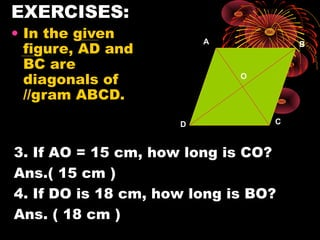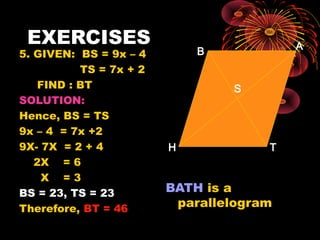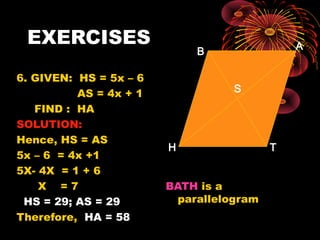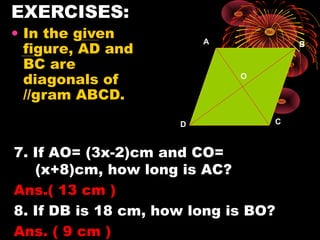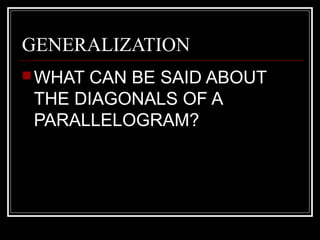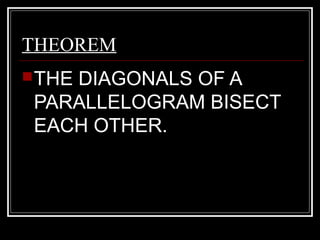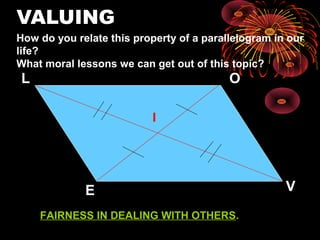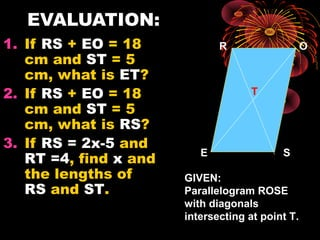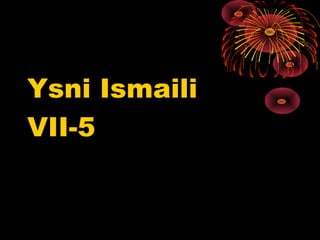1 de 288 5 Trapezoid And Kitesguestc175586Properties of ParallelogramsMelchor CachuelaTrapezoidsDarcy fajardoProperties of ParallelogramCipriano De Leontrapezoid and its propertiesAidrelyn Namuco### Properties of a parallelogram

1. CHAPTER 4. QUADRILATERALS PARALLELOGRAM AND ITS PROPERTIES
2. Define the following:  Midpoint of a segment ( a point on the segment that divides the segment into two congruent parts)  Congruent segments (are two segments whose measures are equal )  Bisector of an angle ( a ray that divides an angle into two congruent measures)
3. When are two triangles congruent?When are two triangles congruent? If two triangles are congruent,If two triangles are congruent, how many pairs of congruenthow many pairs of congruent parts can be shown?parts can be shown? Name these.Name these. CORRESPONDING SIDES FG ≅ XB GH ≅ BM FH ≅ XM CORRESPONDING ANGLES ∠ F ≅ ∠X ∠ G ≅ ∠B ∠ H ≅ ∠M
4. What are some ways to proveWhat are some ways to prove congruent triangles?congruent triangles? SSS CongruenceSSS Congruence PostulatePostulate SAS CongruenceSAS Congruence PostulatePostulate ASA CongruenceASA Congruence PostulatePostulate SAA CongruenceSAA Congruence TheoremTheorem Congruence for RightCongruence for Right TrianglesTriangles Hyl CongruenceHyl Congruence TheoremTheorem HyA congruenceHyA congruence TheoremTheorem LL CongruenceLL Congruence TheoremTheorem LA CongruenceLA Congruence TheoremTheorem
5. Can the two triangles be provedCan the two triangles be proved congruent? If so, what postulatecongruent? If so, what postulate can be used?can be used? SSS Congruence Postulate
6. Can the two triangles be provedCan the two triangles be proved congruent? If so, what postulatecongruent? If so, what postulate can be used?can be used? SAS Congruence Postulate
7. Can the two triangles be provedCan the two triangles be proved congruent? If so, what postulatecongruent? If so, what postulate can be used?can be used? ASA Congruence Postulate
8. What are some general propertiesWhat are some general properties of a parallelogram?of a parallelogram? The opposite sides are both parallelThe opposite sides are both parallel and congruent.and congruent. C A RE CA // RE; CA ≅ RE CE // RA ; CE ≅ RA
9. In the given parallelogram FACE,In the given parallelogram FACE, what does the segment connectingwhat does the segment connecting opposite vertices represent?opposite vertices represent? F AF A MM E CE C
10. THE DIAGONALS OF ATHE DIAGONALS OF A PARALLELOGRAMPARALLELOGRAM OBJECTIVES:OBJECTIVES: 1.To show that the diagonals of a1.To show that the diagonals of a parallelogram bisect each other.parallelogram bisect each other. 2. To solve problems involving2. To solve problems involving diagonals of a parallelogram.diagonals of a parallelogram.
11. CLASS ACTIVITYCLASS ACTIVITY  PROCEDUREPROCEDURE 1.1. Draw and cutout four parallelograms.Draw and cutout four parallelograms. Construct their diagonals. Let the nameConstruct their diagonals. Let the name of the parallelograms beof the parallelograms be FACEFACE with thewith the diagonals intersecting at pointdiagonals intersecting at point MM.. 2.2. With a ruler, measure the distance fromWith a ruler, measure the distance from the vertex to the point of intersection ofthe vertex to the point of intersection of the two diagonals.the two diagonals. 3.3. Record your observation.Record your observation.
12. Data ( Group 1 )Data ( Group 1 ) FMFM CMCM AMAM EMEM ParallelogramParallelogram 11 ParallelogramParallelogram 2(2(squaresquare)) ParallelogramParallelogram 3(3(rectanglerectangle)) ParallelogramParallelogram 4(4(rhombusrhombus))
13. CRITICAL THINKINGCRITICAL THINKING 1.1. Compare: FM and CM ; AM and EM.Compare: FM and CM ; AM and EM. 2.2. Make a conjecture about the diagonalsMake a conjecture about the diagonals of a parallelogramof a parallelogram F A CE M
14. Guide QuestionsGuide Questions 1.1. In your activity, what can be said aboutIn your activity, what can be said about the length of FM compare to the lengththe length of FM compare to the length of CM? How about the length of EMof CM? How about the length of EM compare to the length of AM?compare to the length of AM? 2.2. What segment that bisects FC?What segment that bisects FC? 3.3. What segment that bisects AE?What segment that bisects AE? 4.4. What can be said about the diagonals ofWhat can be said about the diagonals of a parallelogram?a parallelogram?
15. THEOREMTHEOREM THE DIAGONALS OF ATHE DIAGONALS OF A PARALLELOGRAM BISECTPARALLELOGRAM BISECT EACH OTHER.EACH OTHER.
16. Formal proof STATEMENT 1. Parallelogram FACE, with diagonals FC and AE. 2. FA ≅ CE REASON 1. Given 2. Opposite sides of a //gram are congruent. GIVEN: Parallelogram FACE with diagonals FC and AE PROVE: FM ≅ CM ; AM ≅ EM F A CE M 1 2 3 4 PROOF:
17. Formal proof GIVEN: Parallelogram FACE with diagonals FC and AE PROVE: FM ≅ CM ; AM ≅ EM F A CE M 1 2 3 4 PROOF: • STATEMENT • 3. FA// EC ;FE // AC • 4. ∠1≅ ∠4;∠2 ≅∠3 • 5. ∆FMA ≅ ∆CME • 6. FM ≅ CM • AM ≅ EM • REASON • 3. Definition of//gram • 4. If 2 // lines are cut by a transversal, the alternate interior angles are congruent. • 5. ASA Congruence • 6. CPCTC
18. EXERCISES: • In the given figure, AD and BC are diagonals of //gram ABCD. A B C D O 1. AD = 10 cm, how long is BC? Ans.( 10 cm ) 2. If AB is 30 cm, how long is DC? Ans. ( 30 cm )
19. EXERCISES: • In the given figure, AD and BC are diagonals of //gram ABCD. A B CD O 3. If AO = 15 cm, how long is CO? Ans.( 15 cm ) 4. If DO is 18 cm, how long is BO? Ans. ( 18 cm )
20. EXERCISES 5. GIVEN: BS = 9x – 4 TS = 7x + 2 FIND : BT SOLUTION: Hence, BS = TS 9x – 4 = 7x +2 9X- 7X = 2 + 4 2X = 6 X = 3 BS = 23, TS = 23 Therefore, BT = 46 BATH is a parallelogram S B A TH
21. EXERCISES 6. GIVEN: HS = 5x – 6 AS = 4x + 1 FIND : HA SOLUTION: Hence, HS = AS 5x – 6 = 4x +1 5X- 4X = 1 + 6 X = 7 HS = 29; AS = 29 Therefore, HA = 58 BATH is a parallelogram S B A TH
22. EXERCISES: • In the given figure, AD and BC are diagonals of //gram ABCD. A B CD O 7. If AO= (3x-2)cm and CO= (x+8)cm, how long is AC? Ans.( 13 cm ) 8. If DB is 18 cm, how long is BO? Ans. ( 9 cm )
23. GENERALIZATION WHAT CAN BE SAID ABOUT THE DIAGONALS OF A PARALLELOGRAM?
24. THEOREM THE DIAGONALS OF A PARALLELOGRAM BISECT EACH OTHER.
25. VALUING L O E V I How do you relate this property of a parallelogram in our life? What moral lessons we can get out of this topic? FAIRNESS IN DEALING WITH OTHERS.
26. EVALUATION: 1. If RS + EO = 18 cm and ST = 5 cm, what is ET? 2. If RS + EO = 18 cm and ST = 5 cm, what is RS? 3. If RS = 2x-5 and RT =4, find x and the lengths of RS and ST. R O E S T GIVEN: Parallelogram ROSE with diagonals intersecting at point T.
27. Ysni Ismaili VII-5